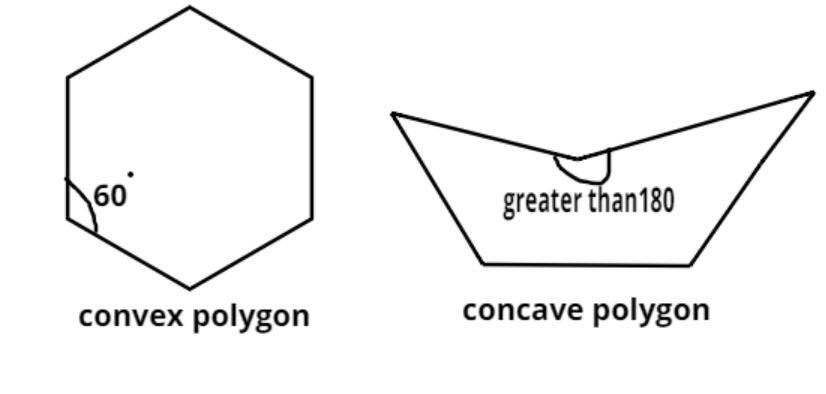QuestionAnswers

# Define Convex polygon and concave polygon.Hint: This is a definition based problem so first let’s understand the meaning of polygon. Polygon is a basic plane figure which consists of n number of line segments forming a closed circuit. Convex in general resembles a figure which is more curved outwards in terms of geometry but however in case of polygon it has a different meaning. Similarly concave in general resembles a figure which is more curved inwards in terms of geometry but however in case of polygon it too has a different meaning.Convex Polygon – A polygon is said to be convex if all the interior angles of the polygon are less than 180 degree. This means that all the vertices of the polygon will point outwards, away from the interior of the shape.
Concave polygon – A polygon is said to be concave if one or more than one interior angle of the polygon is greater than 180 degree.

Note: Whenever we face such types of problems we just need to be clear with the basic dentitions of polygon, concave and convex. Simple diagrammatic representation surely helps in better understanding of these definitions.
View Notes
Concave PolygonConvex PolygonRegular PolygonArea of PolygonPolygon Curve AngleTypes Of PolygonConcave and Convex LensConcave and Convex MirrorsPolygon FormulaPerimeter of a Polygon